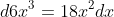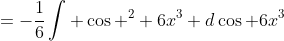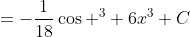## Saturday, 19 May 2012

### Basics of integration. Part III. Ordinary Substitution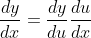Example 1. (Chain rule) Evaluate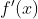where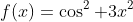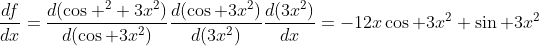Algebrically reading the equation it looks like "multiplying and divising the same thing to the entire function like rationalization and transforming complex number to standard form.

Apply chain rule to a composite function: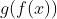. By chain rule we have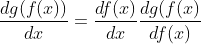If you are not sure about what the second term means, do a substitution u=f(x) and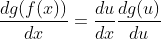.

Now integrate both sides once: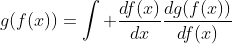(notice that RHS can cancel out each other to give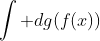which clearly gives the term at LHS.)

We can transform the above equation to another common representation for easier reading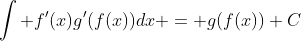.

Theorem 1. Integration by substitution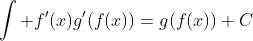.

For those who were not familiar with substitution, you may follow this flowchart:
STEP 1: Identify f'(x) and g(f(x)). In most case in college level this is clear because g(f(x)) is supposed to be the ONLY composite function that can't be easily decomposed.

Example 2a. Identifying composite function Decide which one should be the composite function: i)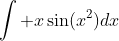, ii)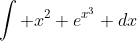iii)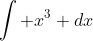For i) and ii)this is clear that there's only one function has hard to break down, that is sin (x^2) and e^(x^3) respectively. For iii), first we know that it's a polynomial, and we know that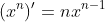, then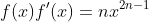, constant does not matter so we have 2n-1 = 3, n = 2. Then we have the composite function to be. Note that we are now applying an exceptional case of the about substitution.

Theorem 2. Exceptional case of substitution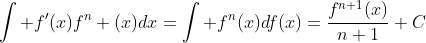where g(x) = x in theorem 1.

STEP 2: Write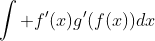in forms of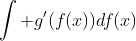.

Note that the constant matters so that we may have to put a constant multiplying the integrand.

Example 2b. Conduct step 2 for the three examples in example 2a.
i)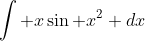. Notice that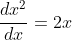, i.e.,, then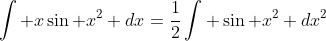ii)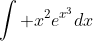. Skip the ordinary form of differentiation and now we get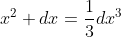, then we haveiii)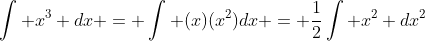STEP 3: Finish the integration. This one should be simple since the function is in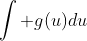where g is easy enough to be integrated as identified.

Example 2c. Finish the integration as shown in example 2b.

i)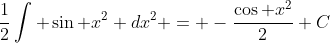ii)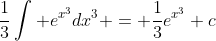iii)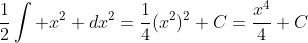Note that we have verified power rule in example (iii).

Example 3. (Verification of power rule) Show that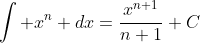for positive odd integer.

For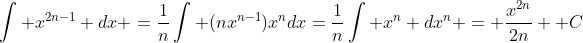.

Sometimes the function will not be as trivial as it shown above. The first extention is about constants sticking in the function.

Theorem 3. (Derivatives on function with constants)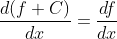where C is a constant.

This is a fact that has been proved in part II, but the one we have to use is:

Corollary 1.. Note that this can be directly proofed as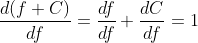.

WARNING. Note that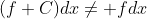! When we put functions outside into the dx stuffs it actually processes an integration and therefore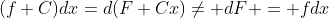.

Therefore it allows us to ignore to do substitution without trouble from constant.

Example 4. Evaluate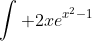We have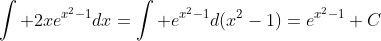because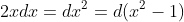. Note that in this example we can take the constant away like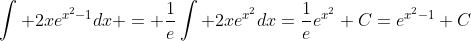.

Another exception from substitution is a linear f(x).

Let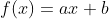,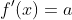.

Consider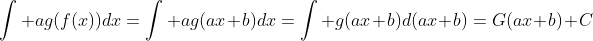.

Theorem 4. (Linear composite functions)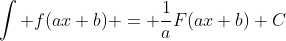Example 5. Evaluate the following integral: i)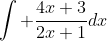ii)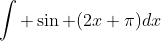i) We have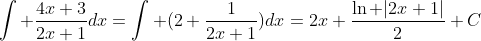because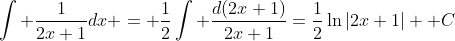.

ii)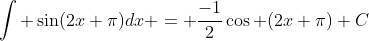, it has the alternative form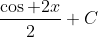by taking the constant away using angle sum formula. We can also take the constant away before integration because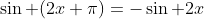which allows us to do the integration easily.

Now we end up this section with two harder example:

Example 6. (Complicated f(x)) Evaluate the integral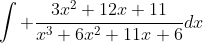.

Noticing that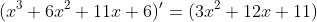we have the integral equal to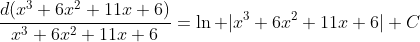.

If there's an alternative method which is by partial fraction which we may discuss later. Noticing that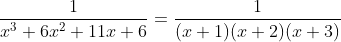we break down the fractions into linear combination of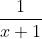,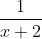and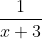, in this case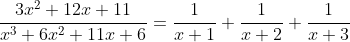(This is another interesting fact concerning polynomial.) Then the integral can be easily evaluated as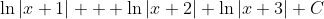which is equivalent to the previous answer.

Example 7. (Multiple composite functions) Evaluate the integral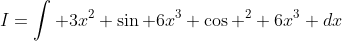.
In this case we have no choice but to use substitution twice.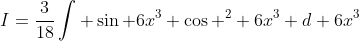because